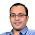### Determinant of Matrix (17-11)

Source: Mailed by Sudeep Kamath (EECS PhD Student, UC Berkeley, EE IITB 2008 Alumnus)

Problem:

A is a 300 x 300 matrix with 17 on the diagonal, and the rest of the entries being 11. What is det (A) ?

Update: (21 June 2014):

Solution posted by Gowtham R (Stanford), Justin Rising, Pavan Bharadwaj, Hansaplatz and gaurushh in comments! Thanks

1.Define M_n as the n x n matrix with diagonal entries all be x and the non-diagonal entries all y. Let D_n be the determinant of M_n and D_n' be the determinant of M_n' where M_n' is obtained from M_n by replacing the (1,1)-th entry by y.

To compute determinants, we only replace (row 1) -> (row 1)-(row 2) for both M_n and M_n'.

Then we have the following recursion:
D_n = (x-y)(D_{n-1}+D_{n-1}')
D_n'=(x-y) D_{n-1}'.
Solving the recursion for D_n gives D_n=(x-y)^{n-1}(x+(n-1)y).
Substituting n=300, x=17,y=11 gives D_{300}=6^300*19*29.

1.Awesome. Thanks. _/\_ :)

2.The easiest way to do this is by working out the eigendecomposition of a general matrix of this form. Consider a matrix of the form aI_n + bJ_n, where I_n is the n x n identity matrix, and J_n is the n x n matrix whose entries are identically one. The eigenvectors are the vector of ones and the basis of the orthogonal complement of the space spanned by the vector of ones. The eigenvalue corresponding to the vector of ones is a + nb, and the eigenvalue corresponding to any other eigenvector is a. The first eigenvalue has multiplicity one and the second has multiplicity n - 1, so the determinant is simply a^(n - 1) * (a + nb).

In this case, a = 6, b = 11 and n = 300, so the determinant is 6^299 * (6 + 300 * 11). We can rewrite that as 6^300 * (1 + 50 * 11). 551 = 19 * 29, so my answer agrees with the previous one.

1.Great solution. Thanks

3.It can be seen as a sum of a matrix with all 11s and a matrix with 6s on the diagonal.

That is the sum of a diagonal matrix and a rank one update to it. You can use http://en.wikipedia.org/wiki/Matrix_determinant_lemma, which gives (and kills the joy in the problem)

Given diagonal element x and off-diagonal y, Det = (x-y)^{n-1}(x+(n-1)y).

1.:-) Works. Thanks

4.First we agree on that it is enough to calculate f(x) = det( I + x N ), where N is matrix with all entries 1. Then by
det(AB) = det (A) det(B), we see that f is a polynomial satisfying f(x) f(y) = f( x + y + nxy) with f(0) = 1. Now we can use standard calculus to solve this functional equation. Of course, we have to argue that f is not the constant function 1. But this is ok because if it was the case, characteristic polynomial of N will have all the coefficient 0 except the leading one. But the trace of N is non zero. So, we get f(x) = 1+ nx

1.In your example, x = 11/6. Answer = det( I + xN )*6 = 6^n*f(x) = 6^n*(1+nx) = 6^n*(1+50*11) = 6^300*551 . Thanks

5.When you do the Singular Value Decomposition (SVD) of matrices of same format but have smaller dimension (Let say n) such as n = 2, n = 3, etc. You can certainly see that the first singular value is 17 + (n-1)*11 and the rest of the singular values are 6. And as the matrix is symmetric, its singular values are equal to its absolute value of eigen values and hence the determinant would be [17+(n-1)*11]*6^(n-1). Putting n = 300 gives the desired result, which is same as the first two comments.

1.